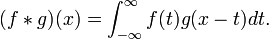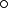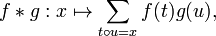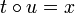# Convolution (mathematics)

In mathematics, convolution is a process which combines two functions on a set to produce another function on the set. The value of the product function depends on a range of values of the argument.

Convolution of real functions by means of an integral are found in probability, signal processing and control theory. Algebraic convolutions are found in the discrete analogues of those applications, and in the foundations of algebraic structures.

## Integral convolutions

The convolution of integrable real functions f and g may be defined as the real function$(f*g)(x) = \int_{-\infty}^\infty f(t) g(x-t) dt .$

Conditions need to be imposed on f and g for this to make sense, such as having compact support or rapid decay at infinity. Other ranges of integration, that is, other domains of definition for the functions involved, may also be used.

## Algebraic convolutions

Let M be a set with a binary operation$\circ$ and R a ring. Let f and g be functions from M to R. The convolution of f and g is a function from M to R$f*g : x \mapsto \sum_{t \circ u = x} f(t)g(u) ,\,$

where the addition and multiplication are those of R. For this sum to make sense it must be finite. If M has the "locally finite" property that for any given x there are only finitely many pairs (t,u) such that$t \circ u = x$, this definition makes sense for any functions f,g. Alternatively, if we restrict to functions of finite support, then no condition on M is needed.

## Transforms

The Fourier transform translates convolution into pointwise multiplication of functions.Some content on this page may previously have appeared on Citizendium.